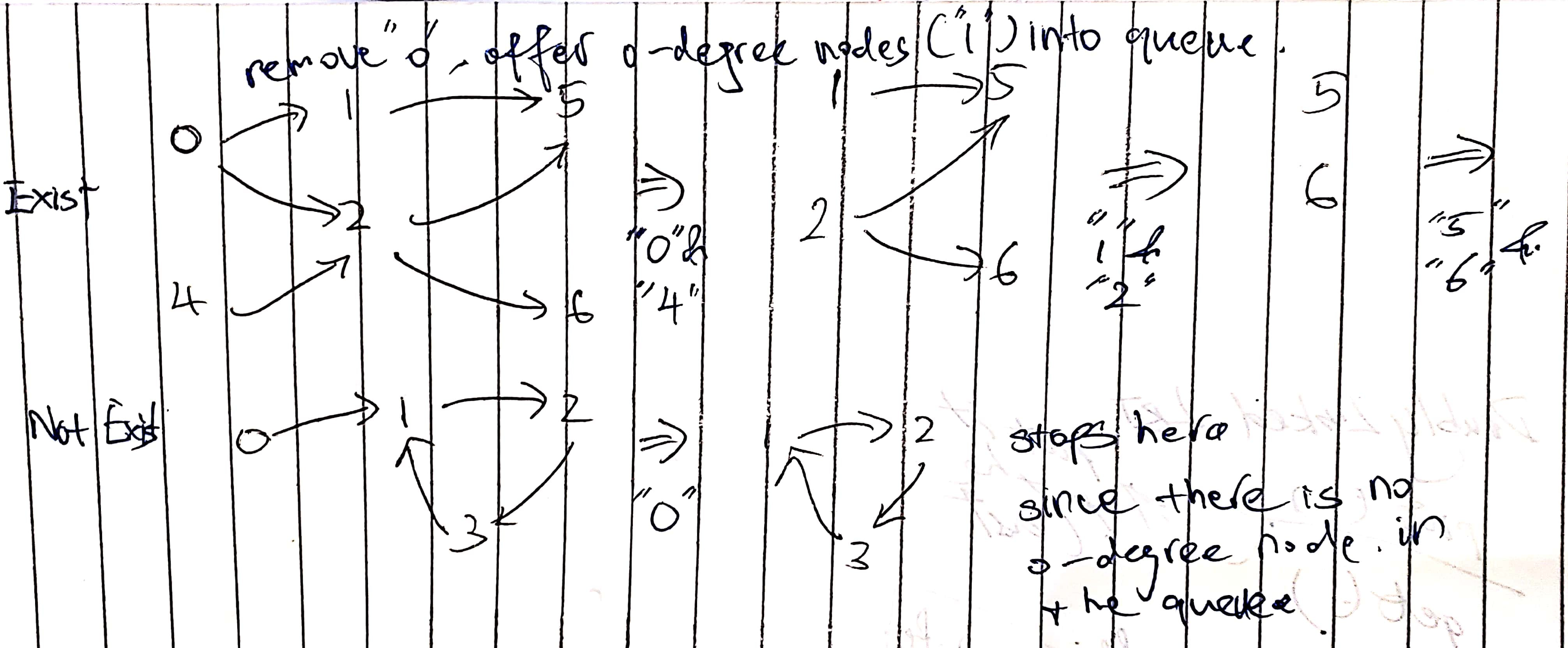# 207. Course Schedule I & II

Reference: Problem I, Problem II
Difficulty: Medium

## Problem I

There are a total of n courses you have to take, labeled from 0 to n-1.

Some courses may have prerequisites, for example to take course 0 you have to first take course 1, which is expressed as a pair: [0,1]

Given the total number of courses and a list of prerequisite pairs, is it possible for you to finish all courses?

Note:

• The input is represented by a list of edges, not adjacency matrices or list.
• You may assume that there are no duplicate edges in the input prerequisites.

Example:

### DFS (Cycle Detection)

This problem is equivalent to finding if a cycle exists in a directed graph. If a cycle exists, no topological ordering exists and therefore it will be impossible to take all courses.

My mistake: Using only true/false marked array does not solve the problem. We need more information because we are not dealing with a problem like finding a cycle in a path.

marked array has three states:

1. If node v has not been visited, then mark it as 0.
2. If node v is being visited, then mark it as -1. If we find a vertex marked as -1 in DFS, then there is a ring.
3. If node v has been visited, then mark it as +1. If a vertex was marked as -1, then no ring contains v or its successors.

Note: First, we convert the input to a graph represented by an adjacency list (gain efficiency).

Time: $O(V + E)$
Space: $O(V)$

### BFS (Topological Sort)

The problem can be reduced to the one that finds if there is a topological sort in the graph (also equivalent to the one that finds if the graph is acyclic).

There are two ways of finding a topological sort: Reversed DFS and BFS. We pick BFS at this time.

The basic idea is that we add all nodes with 0 degrees into a queue, and do BFS from these nodes. They are the starting nodes in a graphs.

Each time we poll a node from the queue, we decrease all its neighbors’ in-degree by one. This is like deleting the node from the graph and removing all its outgoing edges. If a topological sort exists, we can remove the whole graph!

The whole process takes $O(V)$ steps in total if there is a topological sort.So we need a count variable to check how many steps we have along this process. If it finally reduces to 0, then there is a topological sort.

Time: $O(V + E)$
Space: $O(V)$

## Problem II

### DFS (Reversed Post-Order Traversal)

Based on the version of cycle detection, we can update it to construct a topological ordering.

1. Perform a DFS traversal from every vertex (any order) in the graph, not clearing markings between traversals (means not traversing marked vertices).
2. Record DFS post-order along the way (add to list when backtracking).
3. Topological ordering is the reverse of the post-order.

Time: $O(V + E)$
Space: $O(V)$

### BFS (Simpler)

Return a node list in topological ordering. Use BFS. Add to the list when a node is polled from the queue.

Note:

• Base case! When there is no prerequisite, return a list of all nodes.
• When there is no topological sort, return an empty array.

Time: $O(V + E)$
Space: $O(V)$

CommentJunhao Wang
a software engineering cat